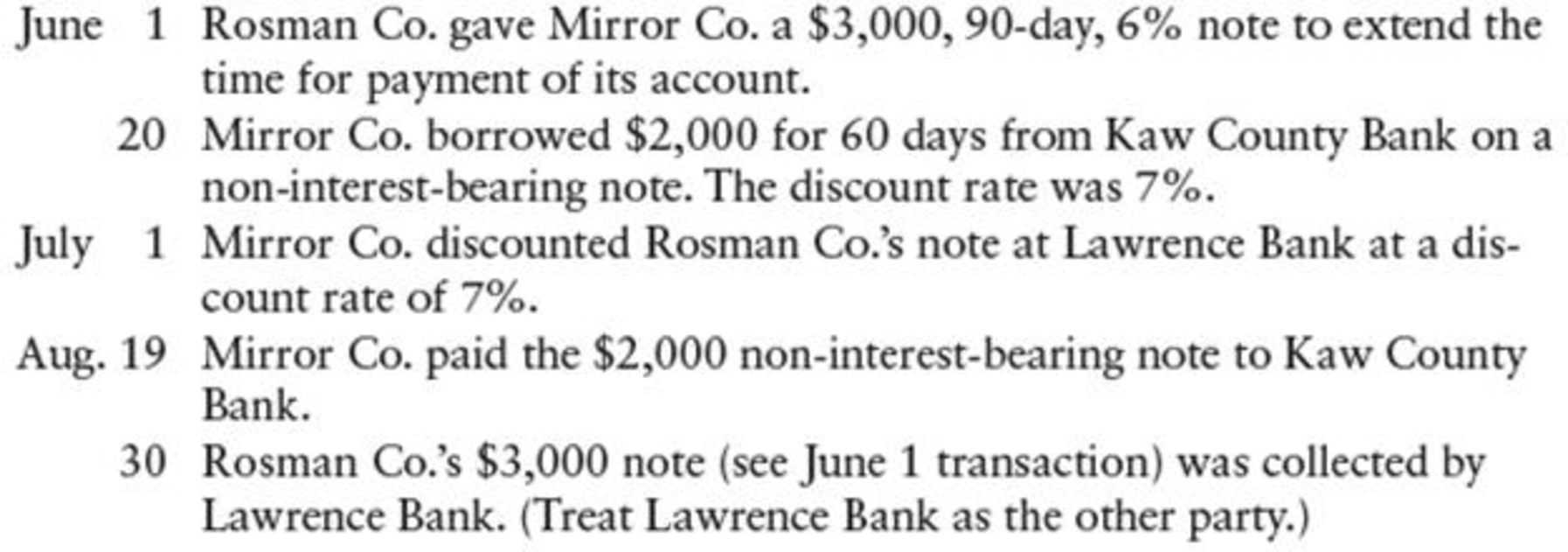Chapter 17, Problem 1CP

Chapter
Section
Textbook Problem

REQUIREDPrepare general journal entries on the books of both Mirror Co. and the other party (either Rosman Co., Kaw County Bank, or Lawrence Bank) for each of the following transactions:To determine

Prepare journal entries in the books of both Company M, and other parties like Company R, KC Bank, and Bank L for each of the following transactions.

Explanation

Prepare journal entries in the books of Company M.

 Date Account titles and Explanation Debit Credit June 1 Notes receivable $3,000 Accounts receivable$3,000 (To record received note to settle account) June 20 Cash $1,976.67 Discount on notes payable (1)$23.33 Notes payable $2,000 (To record note issued for bank loan) July 1 Cash (2)$3,009.47 Notes receivable $3,000 Interest revenue (3)$9.47 (To record discounted note to Company R) August 19 Notes payable $2,000 Interest expense$23.33 Cash $2,000 Discount on notes payable$23.33 (To record note paid at maturity) August 30 No journal entry required

Table (1)

Working notes:

(1) Calculate discount on notes payable.

Discount on notes payable =Notes payable×Interest rate×Time period=$2,000×7%×60360=$23.33

(2) Calculate cash proceeds.

Cash proceeds =Maturity value Discount amount (Difference in time period)=($3,000+($3,000×6%×90360))($3,045×7%×60360)=$3,045$35.53=$3,009.47

(3) Calculate interest revenue.

Interest revenue =Cash proceedsNotes receivable= $3,009.47$3,000=$9.47 As on 1st June: • Notes receivable is a current asset, and it is increased. Therefore, debit notes receivable account for$3,000.
• Accounts receivable is a current asset, and it is decreased. Therefore, credit accounts receivable account for $3,000. As on 20th June: • Cash is a current asset, and it is increased. Therefore, debit cash account for$1,976.67.
• Discount on notes payable is a contra liability, and it is increased. Therefore, debit discount on notes payable account for $23.33. • Notes payable is a current liability, and it is increased. Therefore, credit notes payable account for$2,000.

As on 1st July:

• Cash is a current asset, and it is increased. Therefore, debit cash account for $3,009.47. • Notes receivable is a current asset, and it is decreased. Therefore, credit notes receivable account for$3,000.
• Interest revenue is a component of stockholders’ equity, and it increases revenue accounts. Therefore, credit interest revenue account for $9.47. As on 19th August: • Notes payable is a current liability, and it is decreased. Therefore, debit notes payable account for$2,000

Still sussing out bartleby?

Check out a sample textbook solution.

See a sample solution

The Solution to Your Study Problems

Bartleby provides explanations to thousands of textbook problems written by our experts, many with advanced degrees!

Get Started